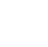Back to Chapter
C++ Setting UpC++ IntroductionNo items found.
C++ BasicsNo items found.
C++ Control StatementsNo items found.
C++ Logical OperatorsNo items found.
C++ Procedural ProgrammingNo items found.
C++ Structural ProgrammingNo items found.
C++ Implementation of OOPSNo items found.
C++ Arrays and VectorsNo items found.
C++ Error HandlingNo items found.
C++ File HandlingNo items found.

# C++ Basic Mathematical Problems

Now let us apply what we've learned and make simple programs to perform basic mathematical operations. Don't just copy-paste the code try writing the code on your own use these codes as a reference. In these examples I've used only 2 inputs, try to solve the same problems by taking multiple inputs.

## Questions

1. Write a program to Prompt the user to input 2 values and display the difference.
2. Write a program to prompt the user to input 2 values and display the sum.
3. Write a program to prompt the user to input 2 values and display the product.
4. Write a program to prompt the user to input 2 variables and display the quotient and remainder.
5. Write a program to prompt the user to input marks in 3 subjects( maths, physics, and chemistry) and display the average marks.

## Solutions:

``` #include using namespace std; main() { int a; int b; cout<<"enter the value of a:"; cin>>a;//the value entered by the user is stored in the 'a' container cout<<"enter the value of b:"; cin>>b;//the value entered by the user is stored in the 'b' container cout<<"the difference is:"<```
``` Output: enter the value of a:5 enter the value of b:9 the difference is:-4 ```

`Answer 2`

``` #include using namespace std; main() { int a; int b; cout<<"enter the value of a:"; cin>>a;//the value entered by the user is stored in the 'a' container cout<<"enter the value of b:"; cin>>b;//the value entered by the user is stored in the 'b' container cout<<"the sum is:"<```
``` Output: enter the value of a:5 enter the value of b:9 the sum is:14 ```

`Answer 3`

``` #include using namespace std; main() { int a; int b; cout<<"enter the value of a:"; cin>>a;//the value entered by the user is stored in the 'a' container cout<<"enter the value of b:"; cin>>b;//the value entered by the user is stored in the 'b' container cout<<"the product is:"<```
``` Output: enter the value of a:5 enter the value of b:9 the product is:45 ```

`Answer 4`

``` #include using namespace std; main() { int a; int b; cout<<"enter the value of a:"; cin>>a;//the value entered by the user is stored in the 'a' container cout<<"enter the value of b:"; cin>>b;//the value entered by the user is stored in the 'b' container cout<<"the quotient is:"<```
`Modulus operator(%) is used for finding the remainder when the number on the left side of the operator is divided by the number on the right side. In this example, 18 is completely divisible by therefore, the remainder is zero.`
``` Output: enter the value of a:18 enter the value of b:9 the quotient is:2 the remainder is:0 ```
`Note that division is possible only if a is greater than b, in the next chapter we will learn how we can resolve this problem and divide any 2 numbers given to us using conditional statements and relational operators.`

`Answer 5`

``` #include using namespace std; main() { double maths; double physics; double chemistry; cout<<"enter the marks in maths:"; cin>>maths; cout<<"enter the marks in physics:"; cin>>physics; cout<<"enter the marks in chemistry:" int avg = (maths+physics+chemistry)/3;//we can assign values after operation on 2 variables to a different variable cout<<"the quotient is:"<```
``` Output: enter the value of marks in maths:96 enter the value of marks in physics:75 the average is:85.5 ```

`If you successfully solve these problems, congrats!!! You've mastered the basics of coding.`

`‍`

`Menu`
`HomeCoursesEventsCampus Ambassador Program`
`About`
`About UsPrivacy PolicyTerms and ConditionsCancellation and Refund PolicyContact UsCareers`
`Courses`
`Full-Stack Web Dev Industrial Training Program`
`Data Scientist Industrial Training Program`
`DevTown Bytes`
`RoadmapsCheatsheetsVideosBlogs`
``` ```
``````
```Ask queriesContact Us on WhatsappHi, How Can We Help You? ```